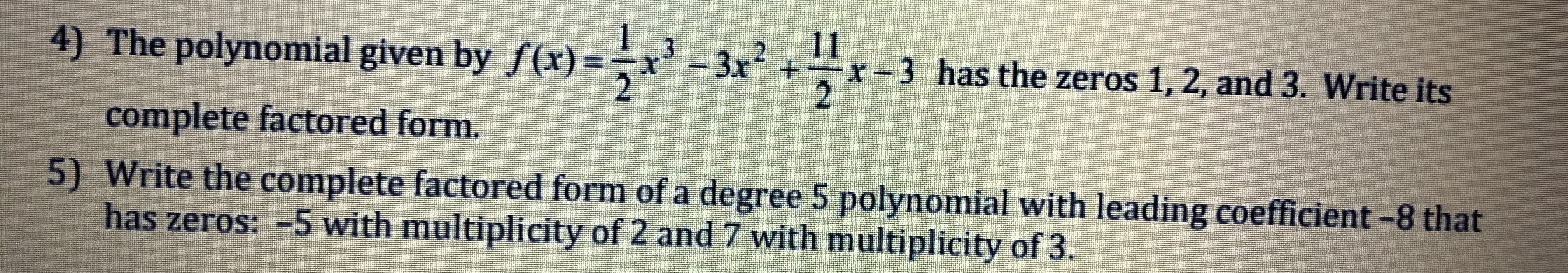4) The polynomial given by f(x)x2+r-3 has the zeros 1, 2, and 3. Write itscomplete factored form.5) Write the complete factored form of a degree 5 polynomial with leading coefficient -8 thathas zeros: -5 with multiplicity of 2 and 7 with multiplicity of 3.

Question

For #5help_outlineImage Transcriptionclose4) The polynomial given by f(x) x2+r-3 has the zeros 1, 2, and 3. Write its complete factored form. 5) Write the complete factored form of a degree 5 polynomial with leading coefficient -8 that has zeros: -5 with multiplicity of 2 and 7 with multiplicity of 3. fullscreen
Step 1

to obtain the expression for the polynomial (satisfying the given condiitons) in the required form

Step 2

A polynomial can have repeated zeros (roos), the number of times the root repeats is called its multiplicity. Here is an example

Step 3

It is clear that if a is a zero of f(x) with multiplicity m, then (x-a...

Want to see the full answer?

See Solution

Want to see this answer and more?

Our solutions are written by experts, many with advanced degrees, and available 24/7

See Solution
Tagged in

Algebra